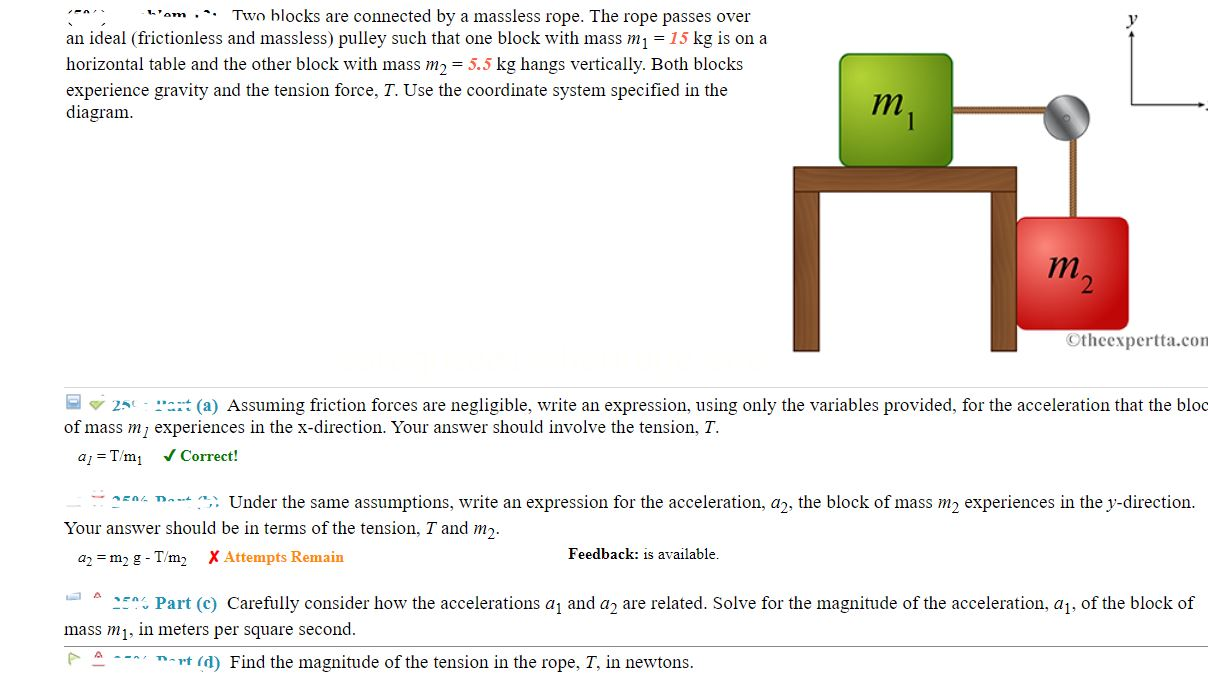# Problem: Two blocks are connected by a massless rope. The rope passes over an ideal (frictionless and massless) pulley such that one block with mass m1 = 15 kg is on a horizontal table and the other block with mass m2 = 5.5 kg hangs vertically. Both blocks experience gravity and the tension force, T. Use the coordinate system specified in the diagram Part (a) Assuming friction forces are negligible, write an expression, using only the variables provided, for the acceleration that the bloc of mass m experiences in the x-direction. Your answer should involve the tension, T Part (b) Under the same assumptions, write an expression for the acceleration, a2, the block of mass m2 experiences in the y-direction Your answer should be in terms of the tension, T and m2. Part (c) Carefully consider how the accelerations a1 and a2 are related. Solve for the magnitude of the acceleration, a1, of the block of 250 mass m1, in meters per square second. Part (d) Find the magnitude of the tension in the rope, T, in newtons.

###### FREE Expert Solution

We'll use the Newton's second law:

$\overline{){\mathbf{\Sigma }}{\mathbf{F}}{\mathbf{=}}{\mathbf{m}}{\mathbf{a}}}$

Part (a)

The force acting on the block m1 in the x-direction is T.

We have, T = m1a1

The acceleration is:

a1 = T/m1

The acceleration that the block of mass m1 experiences in the x-direction is T/m1.

Part (b)

Block of mass m2 experiences T in the positive y-direction and m2g in the negative y-direction.

We have the sum of forces as:

m2g - T = m2a2

100% (476 ratings)###### Problem Details

Two blocks are connected by a massless rope. The rope passes over an ideal (frictionless and massless) pulley such that one block with mass m1 = 15 kg is on a horizontal table and the other block with mass m2 = 5.5 kg hangs vertically. Both blocks experience gravity and the tension force, T. Use the coordinate system specified in the diagramPart (a) Assuming friction forces are negligible, write an expression, using only the variables provided, for the acceleration that the bloc of mass m experiences in the x-direction. Your answer should involve the tension, T

Part (b) Under the same assumptions, write an expression for the acceleration, a2, the block of mass m2 experiences in the y-direction Your answer should be in terms of the tension, T and m2

Part (c) Carefully consider how the accelerations a1 and a2 are related. Solve for the magnitude of the acceleration, a1, of the block of 250 mass m1, in meters per square second.

Part (d) Find the magnitude of the tension in the rope, T, in newtons.